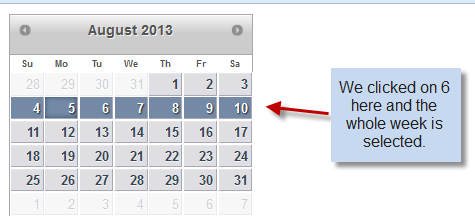Calendar is one of the most commonly used controls if we talk about the web world. The Componentone Wijmo suite offer a large set of useful widgets and the WijCalendar is one of them. Since these widgets are HTML 5 and jQuery based, they offer some really good performance and flexibility. Recently one of our customers wanted to select an entire week when a date is clicked, rather than simply selecting a single date. Here is the final output that we'll achieve through this blog.Though there is no direct way to achieving this, we should not forget to explore the options exposed by the wijcalendar widget and mix it up with normal jQuery. I will try to cover this scenario in this blog article. The first thing we need to take care of is that the selectionMode of the WijCalendar is set to “days”. This would allow a user to select more than one day in the WijCalendar. The idea here is to first identify the day of the week using the date which was clicked upon. Once the day of the week is identified, we can hook it up with the selectedDatesChanged event and select the remaining days of the week conditionally. Let us take a look at the complete code required to implement the approach discussed above:

``````\$(document).ready(function () {
\$("#calendar1").wijcalendar({
showWeekNumbers: false,
selectionMode: { days: true },
selectedDatesChanged: function (e, args) {
var d = \$(this).wijcalendar("getSelectedDate");
var date = new Date(d);
var day = date.getDay();
if (day === 0) {
for (var i = 0; i <= 6; i++) {
\$("#calendar1").wijcalendar("selectDate", new Date(date).setDate(date.getDate() + i));
}
}
else if (day === 1) {
for (var i = -1; i <= 5; i++) {
\$("#calendar1").wijcalendar("selectDate", new Date(date).setDate(date.getDate() + i));
}
}
else if (day === 2) {
for (var i = -2; i <= 4; i++) {
\$("#calendar1").wijcalendar("selectDate", new Date(date).setDate(date.getDate() + i));
}
}
else if (day === 3) {
for (var i = -3; i <= 3; i++) {
\$("#calendar1").wijcalendar("selectDate", new Date(date).setDate(date.getDate() + i));
}
}
else if (day === 4) {
for (var i = -4; i <= 2; i++) {
\$("#calendar1").wijcalendar("selectDate", new Date(date).setDate(date.getDate() + i));
}
}
else if (day === 5) {
for (var i = -5; i <= 1; i++) {
\$("#calendar1").wijcalendar("selectDate", new Date(date).setDate(date.getDate() + i));
}
}
else if (day === 6) {
for (var i = -6; i <= 0; i++) {
\$("#calendar1").wijcalendar("selectDate", new Date(date).setDate(date.getDate() + i));
}
}
}
});
});
``````

So we are all set. Just throw this jQuery code on your WijCalendar page and you will be able to select the whole week simply by clicking on any date. A sample application demonstrating this implementation can be downloaded using the following link: Download Sample HTML file.